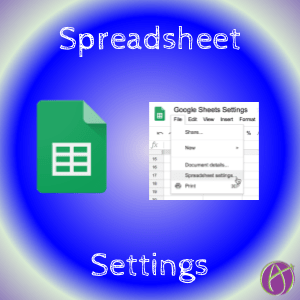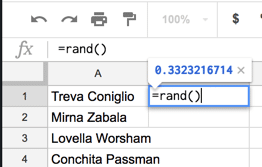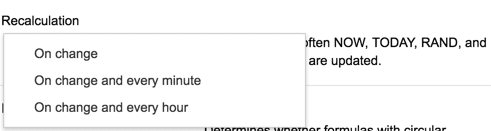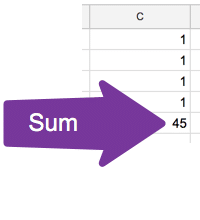# Check Your Settings

It’s probably not something you’ve given a lot of thought to, but there are settings in Google Sheets. As a rule of thumb, I recommend always looking through any program’s settings and considering each setting.

## Time Zone

The setting on the General tab that is most relevant is probably “Time zone.” I have had people message me frustrated that some of the spreadsheet templates I have were calculating in Pacific time when they are not Pacific (I am!).## Calculation Tab

### Recalculation

I use =Rand() a lot in my spreadsheets. This allows me to assign each student a random number. Every time I make a change in the spreadsheet, the random number is updated (changed).You can change the settings so that the recalculation happens not only when there are any edits (changes) to the spreadsheet, but also every minute or every hour also.### Iterative Calculation

Spreadsheets do not like circular formulas. For example if in cell C5 I type the formula =SUM(C1:C5) then I am trying to add the sum to the sum … which makes the spreadsheet very confused and grumpy. Since “Iterative calculation” is turned off you will get an error (#REF) when you have a circular dependency.If you turn ON “Iterative calculation” (and I’ll be honest I have no clue why you would want to do that) it will do your formula a set number of times. Notice in the screenshot below that my formula in C5 is =SUM(C1:C5) and the sum says 45. This is because when you turn on “Iterative calculation” it asks you how many times to do the circular reference. The default is 50. So 50 times it added up the sum of C1 to C5, which includes the sum. It’s a fun math problem to think about but the reality is you do not need that so don’t worry about the math.## Spreadsheet Settings NOT Sheets Settings

It is important to point out that the settings are for that spreadsheet NOT for Sheets. This means they are not global settings. If you change the settings the will not be reflected in other spreadsheets.